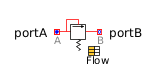Sum - MapleSim Help

Sum

Compute the scaled sumDescription The Sum component computes the scaled sum of the elements of the input vector.Equations $y={k}_{1}{u}_{1}+{k}_{2}{u}_{2}+\cdots +{k}_{{n}_{\mathrm{in}}}{u}_{{n}_{\mathrm{in}}}$Connections

 Name Description Modelica ID $u$ Real input vector u $y$ Real output signal yParameters

 Name Default Units Description Modelica ID $k$ $\mathrm{ones}\left({n}_{\mathrm{in}}\right)$ Real vector of coefficients k ${n}_{\mathrm{in}}$ $1$ Dimension of $u$ ninModelica Standard Library The component described in this topic is from the Modelica Standard Library. To view the original documentation, which includes author and copyright information, click here.